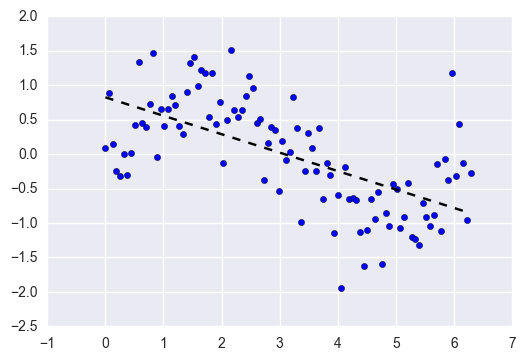Overheard after class: “doesn’t the Bias-Variance Tradeoff sound like the name of a treaty from a history documentary?”

Ok, that’s fair… but it’s also one of the most important concepts to understand for supervised machine learning and predictive modeling.

Unfortunately, because it’s often taught through dense math formulas, it’s earned a tough reputation.

But as you’ll see in this guide, it’s not that bad. In fact, the Bias-Variance Tradeoff has simple, practical implications around model complexity, over-fitting, and under-fitting.## Supervised Learning

The Bias-Variance Tradeoff is relevant for supervised machine learning - specifically for predictive modeling. It's a way to diagnose the performance of an algorithm by breaking down its prediction error.

In machine learning, an algorithm is simply a repeatable process used to train a model from a given set of training data.

As you might imagine, each of those algorithms behave very differently, each shining in different situations. One of the key distinctions is how much bias and variance they produce.

There are 3 types of prediction error: bias, variance, and irreducible error.

Irreducible error is also known as "noise," and it can't be reduced by your choice in algorithm. It typically comes from inherent randomness, a mis-framed problem, or an incomplete feature set.

The other two types of errors, however, can be reduced because they stem from your algorithm choice.

## Error from Bias

Bias is the difference between your model's expected predictions and the true values.

That might sound strange because shouldn't you "expect" your predictions to be close to the true values? Well, it's not always that easy because some algorithms are simply too rigid to learn complex signals from the dataset.

Imagine fitting a linear regression to a dataset that has a non-linear pattern:No matter how many more observations you collect, a linear regression won't be able to model the curves in that data! This is known as under-fitting.

## Error from Variance

Variance refers to your algorithm's sensitivity to specific sets of training data.

High variance algorithms will produce drastically different models depending on the training set.

For example, imagine an algorithm that fits a completely unconstrained, super-flexible model to the same dataset from above:As you can see, this unconstrained model has basically memorized the training set, including all of the noise. This is known as over-fitting.

It's much easier to wrap your head around these concept if you think of algorithms not as one-time methods for training individual models, but instead as repeatable processes.

Let's do a thought experiment:

1. Imagine you've collected 5 different training sets for the same problem.
2. Now imagine using one algorithm to train 5 models, one for each of your training sets.
3. Bias vs. variance refers to the accuracy vs. consistency of the models trained by your algorithm.

We can diagnose them as follows.Low variance (high bias) algorithms tend to be less complex, with simple or rigid underlying structure.

• They train models that are consistent, but inaccurate on average.
• These include linear or parametric algorithms such as regression and naive Bayes.

On the other hand, low bias (high variance) algorithms tend to be more complex, with flexible underlying structure.

• They train models that are accurate on average, but inconsistent.
• These include non-linear or non-parametric algorithms such as decision trees and nearest neighbors.

This tradeoff in complexity is why there's a tradeoff in bias and variance - an algorithm cannot simultaneously be more complex and less complex.

*Note: For certain problems, it's possible for some algorithms to have less of both errors than others. For example, ensemble methods (i.e. Random Forests) often perform better than other algorithms in practice. Our recommendation is to always try multiple reasonable algorithms for each problem.

## Total Error

To build a good predictive model, you'll need to find a balance between bias and variance that minimizes the total error.

Total Error = Bias^2 + Variance + Irreducible Error

Machine learning processes find that optimal balance:A proper machine learning workflow includes:

• Separate training and test sets
• Trying appropriate algorithms (No Free Lunch)
• Fitting model parameters
• Tuning impactful hyperparameters
• Proper performance metrics
• Systematic cross-validation

Finally, as you might have already concluded, an optimal balance of bias and variance leads to a model that is neither overfit nor underfit:This is the ultimate goal of supervised machine learning - to isolate the signal from the dataset while ignoring the noise!